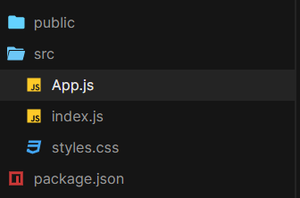# How to improve slow React application rendering ?

• Last Updated : 18 Jun, 2021

ReactJS is a JavaScript library for building User Interfaces. It is a declarative, efficient, and flexible JavaScript library that is maintained by Facebook.

What is Rendering?

React uses the concept of Virtual DOM. All the elements in React are rendered using the render method in which we have to provide an id of an element (mostly div) to be rendered. Every element in React is immutable. Hence, to Update the element, we have to call the Render method again. Please refer here for more details.

What is Slow React Application Rendering?

Slow React application rendering as its name suggests is a small or large delay in rendering elements. There may be multiple reasons for that. This should be avoided.

Methods for improving Slow React Application Rendering:

In the following approach, we will see how hooks can be used effectively to avoid slow rendering. Hooks can play a major role in reducing the number of re-renders. Remember re-render happens whenever there is a change in a state component. It causes the render method to call again and then react updates only a portion that needs to be updated by comparing copies of Virtual DOM.

Following is an example of how useMemo hook comes to the rescue if there is some large calculation in a function.

Creating React Application

• Step 1: Create a React application using the following command:

`npx create-react-app useMemo `
• Step 2: After creating your project folder i.e. useMemo, move to it using the following command:

`cd useMemo`

Project Structure: It will look like the following.Example1: Without using useMemo Hook

## App.js

 `import { useState } from ``"react"``;``import ``"./App.css"``;`` ` `export ``default` `function` `App() {`` ` `  ``// 1st counter state``  ``const [counter1, setCounter1] = useState(0);`` ` `  ``// 2nd counter state``  ``const [counter2, setCounter2] = useState(0);`` ` `  ``// Sample Heavy Calculation Function``  ``const heavyCalculation = () => {``    ``let i = 0;``    ``for` `(let outer = 0; outer < 10000; outer++) {``      ``for` `(let temp = 0; temp < 10000; temp++) {``        ``while` `(i < 10000) i++;``      ``}``    ``}``    ``return` `counter1 % 2 === 0 ? ``true` `: ``false``;``  ``};`` ` `  ``return` `(``    ``
``      ``{heavyCalculation()``        ``? `Counter is even ``with` `value \${counter1}```        ``: `Counter is odd ``with` `value \${counter1}`}``      ``
``      `` {``          ``setCounter1(counter1 + 1);``        ``}}``      ``>``        ``Increment counter1``      ````      ``
``      ``Counter 2 is {counter2}``      ``
``      `` {``          ``setCounter2(counter2 + 1);``        ``}}``      ``>``        ``Increment counter2``      ````    ``
``  ``);``}`

Step to Run Application: Run the application using the following command from the root directory of the project:

`npm start`

Output: Now open your browser and go to http://localhost:3000/, you will see the following output:Explanation: In the above output, a heavy function is being called on Rendering. Now even though it is being used for the first counter, but it still causes render due to counter2 slow.

Example 2: Using useMemo Hook

## App.js

 `import { useState, useMemo } from ``"react"``;``import ``"./App.css"``;`` ` `export ``default` `function` `App() {`` ` `  ``// 1st counter state``  ``const [counter1, setCounter1] = useState(0);`` ` `  ``// 2nd counter state``  ``const [counter2, setCounter2] = useState(0);`` ` `  ``// Our custom useMemo Function``  ``const useMemoFunction = useMemo(() => {``    ``let i = 0;``    ``for` `(let outer = 0; outer < 10000; outer++) {``      ``for` `(let temp = 0; temp < 10000; temp++) {``        ``while` `(i < 10000) i++;``      ``}``    ``}``    ``return` `counter1 % 2 === 0 ? ``true` `: ``false``;``  ``}, [counter1]);`` ` `  ``return` `(``    ``
``      ``{useMemoFunction``        ``? `Counter is even ``with` `value \${counter1}```        ``: `Counter is odd ``with` `value \${counter1}`}``      ``
``      `` {``          ``setCounter1(counter1 + 1);``        ``}}``      ``>``        ``Increment counter1``      ````      ``
``      ``Counter 2 is {counter2}``      ``
``      `` {``          ``setCounter2(counter2 + 1);``        ``}}``      ``>``        ``Increment counter2``      ````    ``
``  ``);``}`

Step to Run Application: Run the application using the following command from the root directory of the project:

`npm start`

Output: Now open your browser and go to http://localhost:3000/, you will see the following output:Explanation: In this that heavy functionality is used by useMemo hook containing counter1 as a dependency. Due to this Counter1 still shows delay, but it does not affect the performance of counter2.

My Personal Notes arrow_drop_up